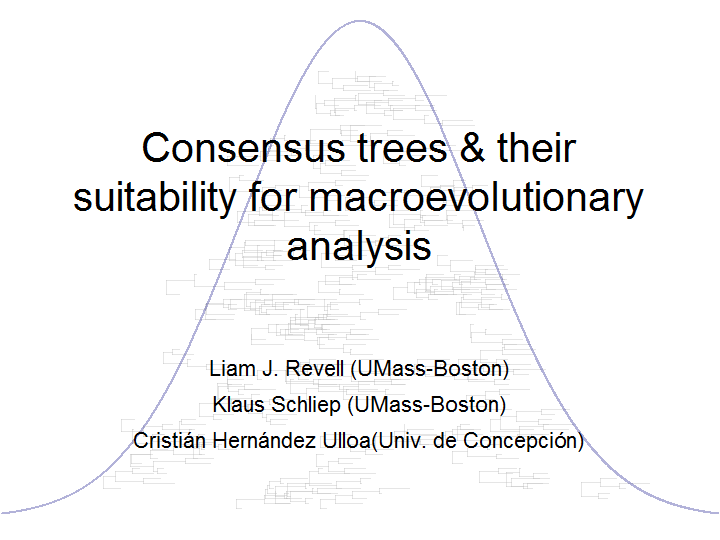## Saturday, April 30, 2016

### Plotting trees under a Gaussian / bell curve

I just submitted my Evolution 2016 talk title, and so I'd thought it might be fun to post the code that I used to generate the title slide image. The method is on the effect of consensus/average tree methods on PCMs, so I decided to (metaphorically) plot some trees under a Gaussian/bell curve. Just in case you should ever care to do the same, here is how I did it:

``````library(phytools)

mt<-phytools:::make.transparent

trees<-rmtree(n=5,N=100)

plot.new()

h<-sapply(trees,function(x) max(nodeHeights(x)))

x0<-10
x<-seq(0,20,by=0.01)
y<-100*exp(-(x-x0)^2/25)
par(mar=rep(0.1,4))
plot(x,y,type="l",axes=FALSE,xlab="",ylab="",col="transparent")

for(i in 1:length(trees)){
x<-runif(n=1)*20
y<-Inf
while(y>100*exp(-((x+h[i]/2)-x0)^2/20)){
x<-runif(n=1)*20
y<-sample(1:90,1)
}
plotTree(trees[[i]],xlim=c(0,20)-x,ylim=c(0,100),
tips=setNames(1:Ntip(trees[[i]])+y,
color=mt("grey",0.4))
}

x0<-mean(par()\$usr[1:2])
x<-seq(par()\$usr,par()\$usr,by=0.01)
y<-100*exp(-(x-x0)^2/25)
lines(x,y,lwd=2,col=mt("navy",0.3))

text("Consensus trees & their\nsuitability for macroevolutionary\nanalysis",
x=mean(par()\$usr[1:2]),y=1.3*mean(par()\$usr[3:4]),cex=3.4)

text("Liam J. Revell (UMass-Boston)",x=mean(par()\$usr[1:2]),
y=0.6*mean(par()\$usr[3:4]),cex=1.8)
text("Klaus Schliep (UMass-Boston)",x=mean(par()\$usr[1:2]),
y=0.6*mean(par()\$usr[3:4])-3.6*strheight("X"),cex=1.8)
text("CristiÃ¡n HernÃ¡ndez Ulloa(Univ. de ConcepciÃ³n)",x=mean(par()\$usr[1:2]),
y=0.6*mean(par()\$usr[3:4])-7.2*strheight("X"),cex=1.8)
``````If we want to export to PDF, we just have to flank the code above with the following lines, and to comment out `plot.new()`. That even allows us to use alternative fonts (although to do so, we first have to install the extrafonts library and take some other preliminary steps that can easily be found online).

``````pdf(file="Evolution-title.pdf",font="Calibri",width=10,height=7.5)
## ... our code
dev.off()
``````

That's all there is to it.